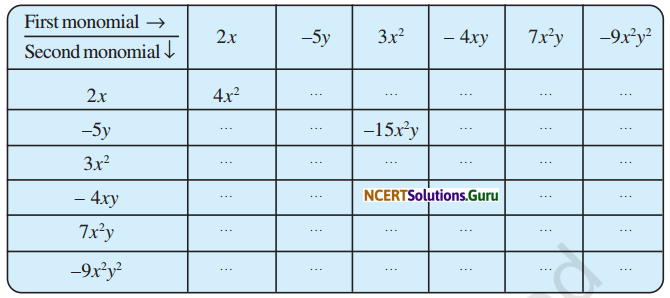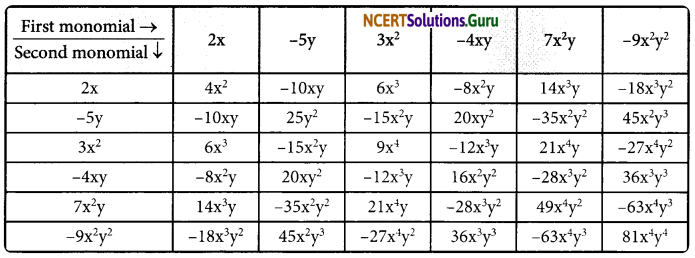# NCERT Solutions for Class 8 Maths Chapter 9 Algebraic Expressions and Identities Ex 9.2

These NCERT Solutions for Class 8 Maths Chapter 9 Algebraic Expressions and Identities Ex 9.2 Questions and Answers are prepared by our highly skilled subject experts.

## NCERT Solutions for Class 8 Maths Chapter 9 Algebraic Expressions and Identities Exercise 9.2

Question 1.
Find the product of the following pairs of monomials
(i) 4, 7p
(ii) -4p, 7p
(iii) -4p, 7pq
(iv) 4p3, – 3p
(v) 4p, 0
Solution:
(i) 4 × 7p
= (4 × 7)p
= 28p

(ii) -4p × 7p
= {(-4) × 7} × (p × p)
= (-28) × p2
= -28p2

(iii) -4p × 7pq
= {(-4) × 7} × {p × (pq)}
= -28 × (p × p × q)
= -28p2q(iv) 4p3 × -3p
= {4 × (-3)} × (p3 × p)
= -12 × (p4)
= -12p4

(v) 4p × 0
= (4 × 0) × p
= 0 × p
= 0

Question 2.
Find the areas of rectangles, with the following pairs of monomials as their lengths and breadths respectively.
(p, q); (10m, 5n); (20x2, 5y2); (4x, 3x2); (3mn, 4np)
Solution:
(i) (p, q)
Area of the rectangle = length × breadth
= p x q
= pq

(ii) (10m, 5n)
Area of the rectangle = length × breadth
= 10m × 5n
= (10 × 5) × (m × n)
= 50 × mn
= 50mn

(iii) 20x2, 5y2
Area of the rectangle = length × breadth
= 20x2 × 5y2
= (20 × 5) × (x2 × y2)
= 100 × x2y2
= 100x2y2(iv) (4x, 3x2)
Area of the rectangle = length × breadth
= 4x × 3x2
= (4 × 3) × (x × x2)
= 12 × x3
= 12x3

(v) (3mn, 4np)
Area of the rectangle = length × breadth
= 3mn × 4np
= (3 × 4) × (mn × np)
= 12 × m × (n × n) × p
= 12 × m × n2 × p
= 12mn2p

Question 3.
Complete the table products.Solution:Question 4.
Obtain the volume of rectangular boxes with the following length, breadth and height respectively.
(i) 5a, 3a2, 7a4
(ii) 2p, 4q, 8r
(iii) xy, 2x2y, 2xy2
(iv) a, 2b, 3c
Solution:
(i) 5a, 3a2, 7a4
Volume of the rectangular box = length × breadth × height
= (5a) × (3a2) × (7a4)
= (5 × 3 × 7) × (a × a2 × a4)
= 105a7(ii) 2p, 4q, 8r
Volume of the rectangular box = Length × Breadth × Height
= 2p × 4q × 8r
= (2 × 4 × 8) × (p × q × r)
= 64pqr

(iii) xy; 2x2y; 2xy2
Volume of the rectangular box = length × breadth × height
= xy × 2x2y × 2xy2
= (1 × 2 × 2) × (x × x2 × x) × (y × y × y2)
= 4 × x4 × y4
= 4x4y4

(iv) a, 2b, 3c
Volume of the rectangular box = length × breadth × height
= a × 2b × 3c
= (1 × 2 × 3) × (a × b × c)
= 6abc

Question 5.
Obtain the product of
(i) xy, yz, zx
(ii) a, -a2, a3
(iii) 2, 4y, 8y2, 16y3
(iv) a, 2b, 3c, 6abc
(v) m, -mn, mnp
Solution:
(i) xy, yz, zx
(xy) × (yz) × (zx)
= (x × x) × (y × y) × (z × z)
= x2 × y2 × z2
= x2y2z2

(ii) a; -a2; a3
(a) × (-a2) × (a3)
= -(a × a2 × a3)
= -a6

(iii) 2, 4y, 8y2, 16y3
(2) × (4y) × (8y2) × (16y3)
= (2 × 4 × 8 × 16) × (y × y2 × y3)
= 1024y6(iv) a, 2b, 3c, 6abc
(a) × (2b) × (3c) × (6abc)
= (2 × 3 × 6) × (a × a) × (b × b) × (c × c)
= 36 × a2 × b2 × c2
= 36a2b2c2

(v) m, -mn, mnp
(m) × (-mn) × (mnp)
= -1 × (m × m × m) × (n × n) × p
= -1 × m3 × n2 × p
= -m3n2p

error: Content is protected !!